# Physical Properties of Engineering Materials

To finalize the material for an engineering product or application, we should have the knowledge of physical properties of materials. The physical properties of a material are those which can be observed without any change of the identity of material. Some of these typical properties of a material are listed below-

• Density
• Specific gravity
• State Change temperatures
• Coefficients of thermal expansion
• Specific Heat
• Latent heat
• Fluidity
• Weld ability
• Elasticity
• Plasticity
• Porosity
• Thermal conductivity
• Electrical Conductivity

## Density of Materials

Density of a material or substance is defined as “the mass per unit volume”. It is represented as the ratio of mass with volume of a material. It is denoted by “ρ”. Its unit in SI system is Kg/m3.
If, m is the mass of material in Kg, V is the volume of materiel in meter3.
Then the Density of material,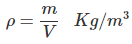## Specific Gravity of Materials

It is defined as the ratio of density of material with respect to density of a reference material or substance. It does not have any unite. Sometimes it is also called as relative density. For gravity calculation generally water is considered as a reference substance.

## State Change Temperatures

Generally a substance has three states called – solid state, liquid state, gaseous state. State change temperature is the temperature at which the substance changes from one state to another state.

State change temperature are of following types-

Melting point-It is the temperature (in oC or K) at which the substance changes from solid state to liquid state.

Boiling point-It is the temperature (in oC or K) at which the substance changes from liquid state to gaseous state.

Freezing point-It is the temperature (in oC or K) at which a liquid changes from liquid to solid state. Theoretically it is equal to the melting point. However, practically there may be observed some difference.

## Coefficient of Thermal Expansion

When a material is heated, it expands, due to which its dimensions change. Coefficient of thermal expansion, represents the expansion in material with increase in temperature. Thermal expansion coefficients are of three types, namely-

Coefficient of Linear Thermal Expansion
The change in length of an object due change in temperature is related by “Coefficient of linear thermal expansion”. It is denoted by “αL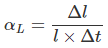Where, ‘l’ is the initial length of object, ‘Δl’ is the change in length, ‘Δt’ is the change in the temperature. Unit of αL is per oC.

Coefficient of Area Thermal Expansion
The change in area of an object due change in temperature is related by “Coefficient of Area thermal expansion”. It denoted by “αA”.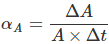Where, ‘l’ is the initial length of object, ‘ΔA’ is the change in length, ‘Δt’ is the change in the temperature. Unit of αA is per oC.

Coefficient of Volume Thermal Expansion
The change in volume of an object due change in temperature is related by “Coefficient of volume thermal expansion”. It denoted by “αV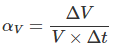Where, ‘l’ is the initial length of object, ‘ΔV’ is the change in length, ‘Δt’ is the change in the temperature. Unit of αA is per oC.

## Specific Heat of Materials

Specific heat of a material is defined as the amount of heat required to increase the temperature of unit mass of material by 1oC. It is denoted by ‘S’.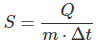Where, m is the mass of material in Kg. Q is the amount of heat given to material in Joule. Δt is rise in temperature. Unit of specific heat in SI system is Joule/Kg oC.

## Latent Heat of Materials

Latent Heat of a material is defined as the amount of heat required/released by change in unit mass of material from one state to another state (Phase change). It is denoted by ‘L’. Latent heat is given by,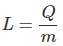Where, ‘Q’ is the amount of heat required/released by material (in joule), ‘m’ is the mass of material (in Kg). Unit of Latent heat in SI system is Joule/Kg.

## Fluidity of Materials

It is a property of material which represents that how easily a material can flow in liquid state. It is the reciprocal to viscosity of liquid material.

## Weld Ability of Materials

It is the property of a material which presents that how easily the two pieces of material can be welded together by applying pressure or heat or both.

## Elasticity of Materials

It is the property of a material by which it regains its original dimensions on removal of load or force.

## Plasticity of Materials

When we keep on increasing the load beyond the limit of elasticity, the material retains it molded state. This property of materials is called plasticity.

## Porosity of Materials

When a material is in melting condition, it contains some dissolved gases within the material. When the material is solidifies, these gases get evaporated and leave behind voids. The Porosity of material represents the quantity of voids in solid materials.

## Thermal Conductivity of Materials

It is the property of a material which represents that how easily the heat can be conducted by a material.
The thermal conductivity of a material can be defined as “the amount of heat transmitted by unit thickness of material normal to the unit area surface in unit time when the temperature gradient across the material piece is unity in steady state condition”.
Its unit in SI system is watts per meter per K.

## Electrical Conductivity of Materials

It is the property of materials which represents that how easily the electricity can be conducted by the material. It is denoted by ‘σ’. It is the reciprocal of resistivity of material. It unit is mho/meter.

Want To Learn Faster? 🎓
Get electrical articles delivered to your inbox every week.
No credit card required—it’s 100% free.